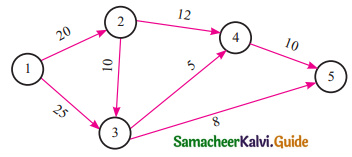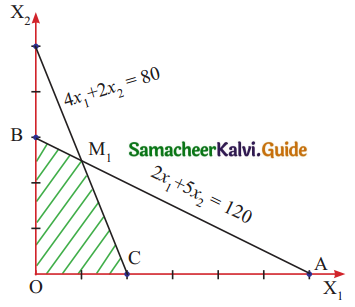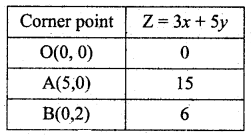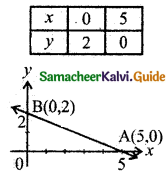Tamilnadu State Board New Syllabus Samcheer Kalvi 11th Business Maths Guide Pdf Chapter 10 Operations Research Ex 10.3 Text Book Back Questions and Answers, Notes.

## Tamilnadu Samacheer Kalvi 11th Business Maths Solutions Chapter 10 Operations Research Ex 10.3

### Samacheer Kalvi 11th Business Maths Operations Research Ex 10.3 Text Book Back Questions and Answers

Question 1.
The critical path of the following network is:(a) 1-2-4-5
(b) 1-3-5
(c) 1-2-3-5
(d) 1-2-3-4-5
(d) 1-2-3-4-5
Hint:
1-2-4-5 ⇒ EFT = 20 + 12 + 10 = 42
1-3-5 ⇒ EFT = 25 + 8 = 33
1-2-3-5 ⇒ EFT = 20 + 10 + 8 = 38
1-2-3-4-5 ⇒ EFT = 20 + 10 + 5 + 10 = 45

Question 2.
Maximize: z = 3x1 + 4x2 subject to 2x1 + x2 ≤ 40, 2x1 + 5x2 ≤ 180, x1, x2 ≥ 0. In the LPP, which one of the following is feasible comer point?
(a) x1 = 18, x2 = 24
(b) x1 = 15, x2 = 30
(c) x1 = 2.5, x2 = 35
(d) x1 = 20.5, x2 = 19
(c) x1 = 2.5, x2 = 35
Hint:
z = 3x1 + 4x2
Let us solve the equations
2x1 + x2 = 40 ………(1)
2x1 + 5x2 = 180 ……….(2)
(1) – (2) ⇒ -4x2 = -140
x2 = 35
We have 2x1 + x2 =40
2x1 + 35 = 40
2x1 = 5
x1 = 2.5Question 3.
One of the conditions for the activity (i, j) to lie on the critical path is:
(a) Ej – Ei = Lj – Li = tij
(b) Ei – Ej = Lj – Li = tij
(c) Ej – Ei = Li – Lj = tij
(d) Ej – Ei = Lj – Li ≠ tij
(a) Ej – Ei = Lj – Li = tij

Question 4.
In constructing the network which one of the following statement is false?
(a) Each activity is represented by one and only one arrow, (i.e) only one activity can connect any two nodes.
(b) Two activities can be identified by the same head and tail events.
(c) Nodes are numbered to identify an activity uniquely. Tail node (starting point) should be lower than the head node (end point) of an activity.
(d) Arrows should not cross each other.
(b) Two activities can be identified by the same head and tail events.Question 5.
In a network while numbering the events which one of the following statement is false?
(a) Event numbers should be unique.
(b) Event numbering should be carried out on a sequential basis from left to right.
(c) The initial event is numbered 0 or 1.
(d) The head of an arrow should always bear a number lesser than the one assigned at the tail of the arrow.
(d) The head of an arrow should always bear a number lesser than the one assigned at the tail of the arrow.

Question 6.
A solution which maximizes or minimizes the given LPP is called:
(a) a solution
(b) a feasible solution
(c) an optimal solution
(d) none of these
(a) a solutionQuestion 7.
In the given graph the coordinates of M1 are(a) x1 = 5, x2 = 30
(b) x1 = 20, x2 = 16
(c) x1 = 10, x2 = 20
(d) x1 = 20, x2 = 30
(c) x1 = 10, x2 = 20
Hint:
4x1 + 2x2 = 80 (or) 2x1 + x2 = 40
2x1 + x2 = 40 ……(1)
2x1 + 5x2 = 120 ……(2)
(1) – (2) ⇒ -4x2 = -80
x2 = 20
But, 2x1 + x2 = 40
2x1 + 20 = 20
x1 = 10

Question 8.
The maximum value of the objective function Z = 3x + 5y subject to the constraints x > 0, y > 0 and 2x + 5y ≤ 10 is:
(a) 6
(b) 15
(c) 25
(d) 31
(b) 15
Hint:
Let 2x + 5y = 10∴ Maximum Value = 15Question 9.
The minimum value of the objective function Z = x + 3y subject to the constraints 2x + y ≤ 20, x + 2y ≤ 20, x > 0 and y > 0 is:
(a) 10
(b) 20
(c) 0
(d) 5
(c) 0
Hint:
O(0, 0) is a comer point.
So Z = 0 + 3(0) = 0
∴ Minimum value is 0

Question 10.
Which of the following is not correct?
(a) Objective that we aim to maximize or minimize
(b) Constraints that we need to specify
(c) Decision variables that we need to determine
(d) Decision variables are to be unrestricted
(d) Decision variables are to be unrestrictedQuestion 11.
In the context of network, which of the following is not correct?
(a) A network is a graphical representation.
(b) A project network cannot have multiple initial and final nodes
(c) An arrow diagram is essentially a closed network
(d) An arrow representing an activity may not have a length and shape
(d) An arrow representing an activity may not have a length and shape

Question 12.
The objective of network analysis is to:
(a) Minimize total project cost
(b) Minimize total project duration
(c) Minimize production delays, interruption and conflicts
(d) All the above
(b) Minimize total project durationQuestion 13.
Network problems have advantage in terms of project:
(a) Scheduling
(b) Planning
(c) Controlling
(d) All the above
(d) All the above

Question 14.
In critical path analysis, the word CPM mean:
(a) Critical path method
(b) Crash projecf management
(c) Critical project management
(d) Critical path management